Disclosure: This post may contain affiliate links, meaning when you click the links and make a purchase, we receive a commission.

# Excel Financial Function Tips – NPV vs XNPV

In this tutorial, you will learn how to calculate the net present value using Excel’s NPV and XNPV Functions.

The NPV (Net Present Value) Function is used to calculate the net present value of an investment by evaluating a provided discount rate, a series of future payments (negative values) and income (positive values). The XNPV Function is used to return the net present value for a schedule of cash flows that are not periodic. The NPV is mostly utilized in capital budgeting in order to give an indication of the profitability of a projected investment. An investment calculation that yields a positive NPV, will more than likely be a profitable investment, whereas an investment calculation that results in a negative NPV will more than likely yield a loss. When one has calculated the NPV value for multiple investments and they all yield positive results, then it is advisable to invest in the project with the highest NPV value.

Read More: VLOOKUP versus INDEX and MATCH versus DGET

## The syntax of the NPV function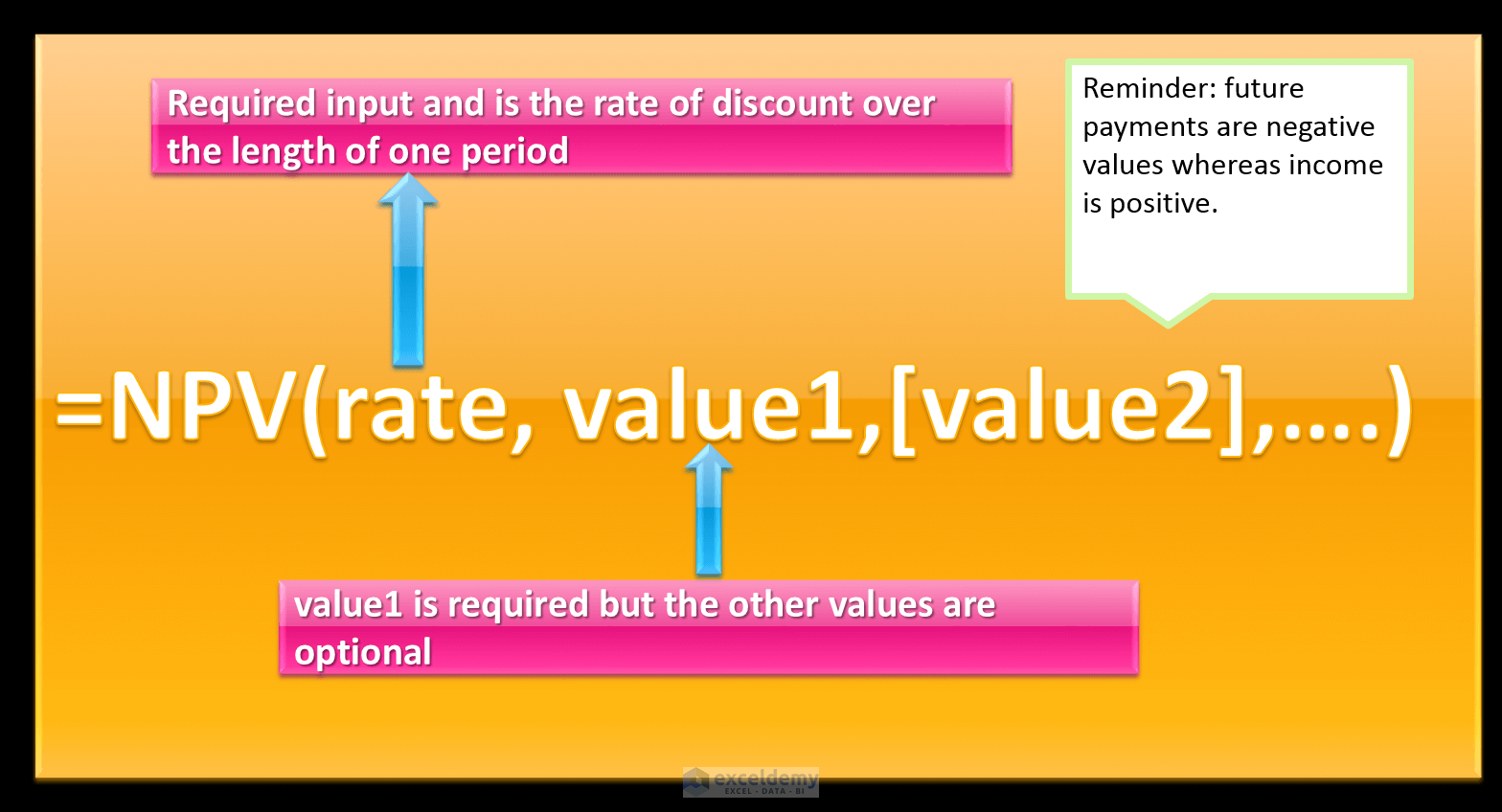## The syntax of the XNPV function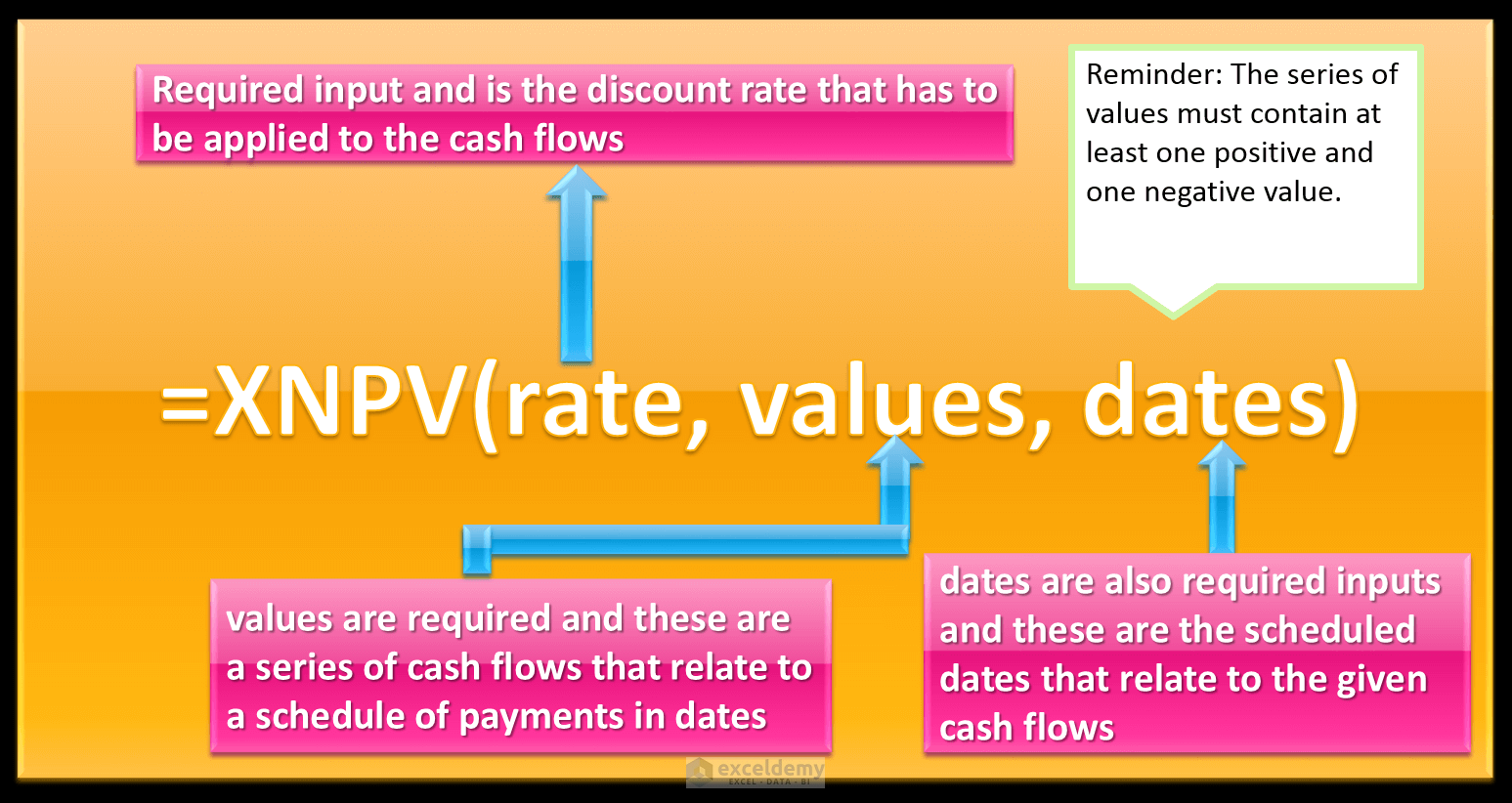So, let’s get started with a simple example to illustrate what NPV and XNPV are used for, and the main difference between them.

Read More: How to Generate Random Numbers in Excel (Ultimate Guide)

## Calculating NPV (Net Present Value) in Excel with NPV Function

The annual discount rate of an investment is 3%, the initial investment cost one year from today is \$19,000. The return from year 1 is \$5,000, year 2 is \$5,500, year 3 is \$5,700 and the return from year 4 is \$6,200.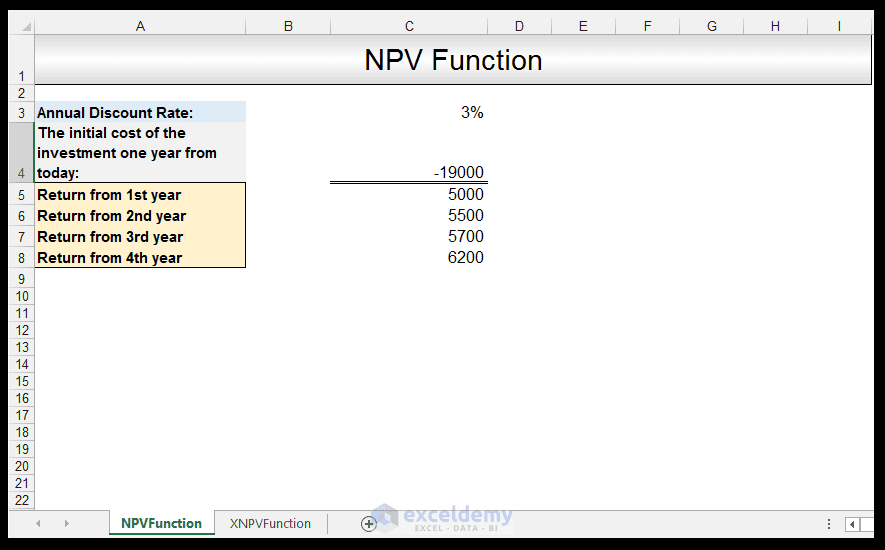1) In order to calculate the NPV, in Cell C9 we input the following formula:

=NPV (C3, C4, C5, C6, C7, C8)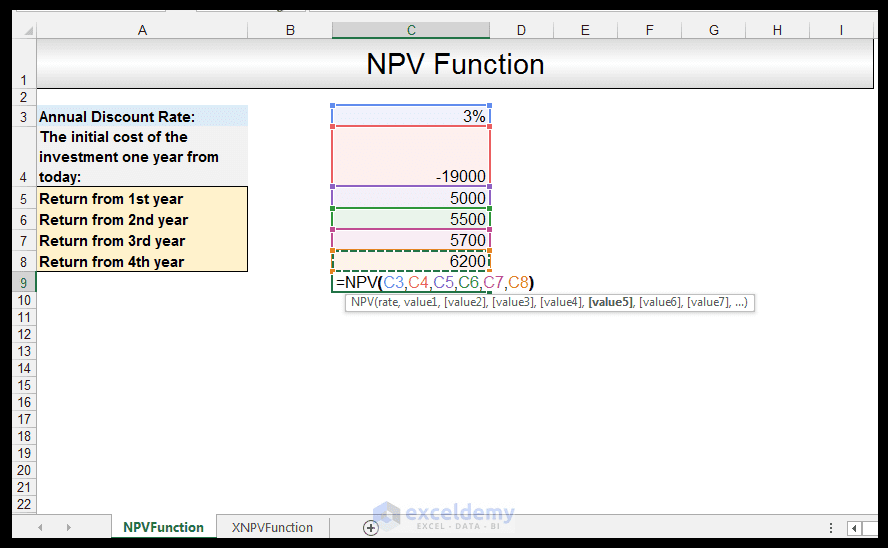2) Upon pressing CTRL-ENTER, a positive value of \$1 712.21 is returned which means that the investment would more than likely be profitable. An investor would thus be advised to consider this investment.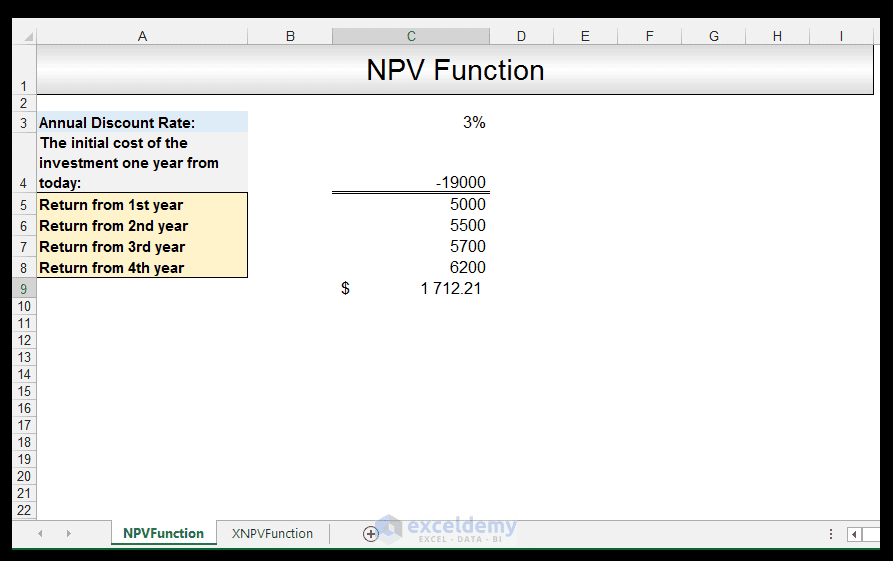A point of value to note is that because the initial payment occurs at the end of the first period, this value is used as value1. If the payment was made at the beginning of the first period, it would not be included in the arguments input to the NPV function and instead added to the result afterward.

Read More: The PV, FV and PDURATION Excel Functions Tutorial

## Calculating Net Present Value in Excel with XNPV Function

The discount rate of an investment is 3%, the initial investment cost made is \$9,000. The return from 2 February 2017 is \$2,000, the return from 7 March 2017 is \$2,200, from 10 September 2017 is \$2,800 and from 3 January 2018 is \$2,900.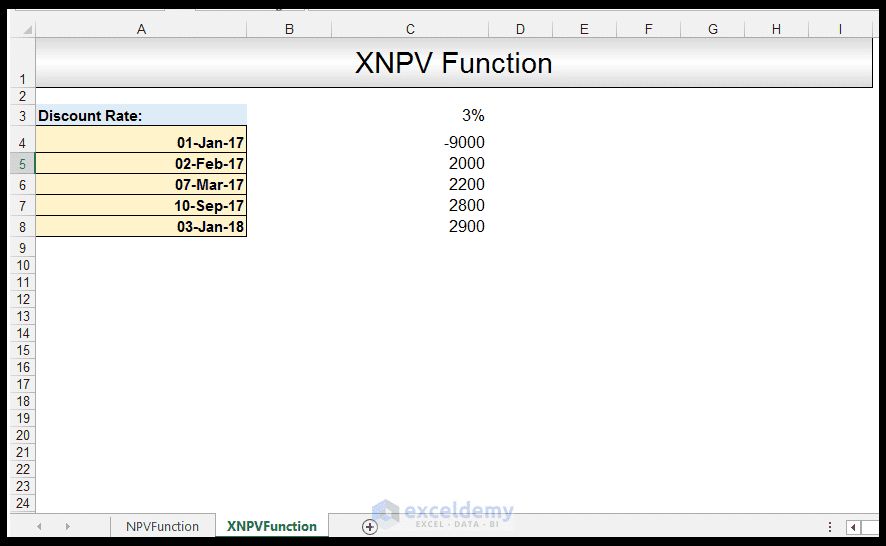1) In order to calculate the XNPV, in Cell C9 we input the following formula:

=XNPV (C3, C4: C8, A4: A8)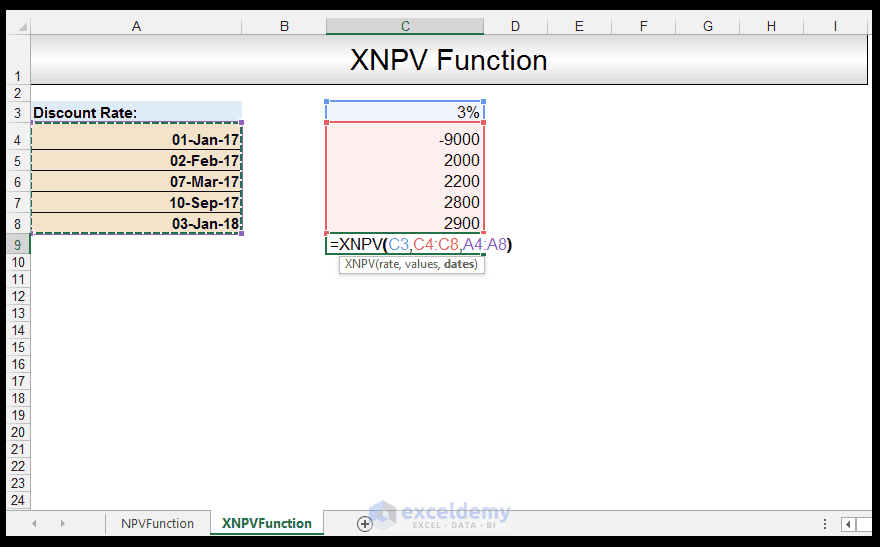2) Upon pressing CTRL-ENTER, a positive value of \$741.79 is returned which means that the investment would more than likely be profitable. An investor would thus be advised to consider this investment.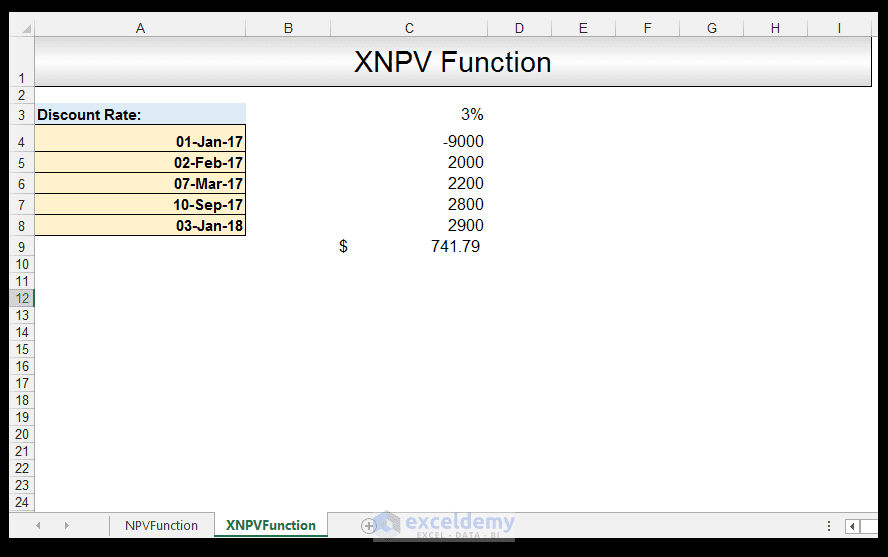NPVandXNPVTutorial

## Conclusion

The main difference between the NPV and the XNPV functions are that the values input in the NPV function are equally spaced, whereas the values input in the XNPV function are not equally spaced and thus related to an exact date.

Please feel free to comment in the attachment and tell us if you use NPV or XNPV to estimate the profitability of an investment.

Learn Excel Online: Top Excel Courses Online

How to Use the Database Functions in Excel

Excel Protection Explained: Worksheet vs. Workbook Level Protection

The NPV Function

The XNPV Function

What is the Net Present Value (NPV) and what is it used for

Taryn is a Microsoft Certified Professional, who has used Office Applications such as Excel and Access extensively, in her interdisciplinary academic career and work experience. She has a background in biochemistry, Geographical Information Systems (GIS) and biofuels. She enjoys showcasing the functionality of Excel in various disciplines.

1. ReplyRAHUL Apr 5, 2017 at 12:14 AM

Thanks a lot Taryn!!!

I m a Finance Student and need this formulas a Lot!!!!

You have very easily explained the concepts.!!!

Looking foreword for more:):):):):):)

• Reply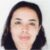Apr 5, 2017 at 7:40 PM

You are most welcome. We are intending to do a financial functions tutorial series so lookout for that 🙂 Best of luck with your studies as well 🙂